# NCERT Solutions for Class 7 Maths Chapter 14 Symmetry

NCERT Solutions for Class 7 Maths Chapter 14 Symmetry- In mathematics, symmetry means that one shape becomes exactly like another when you move(turn, flip or slide) it in some way. In nature, tree-leaves,  beehives, flowers, and in the logo, symbols everywhere you can find various types of symmetrical designs. In this article, you will get CBSE NCERT solutions for class 7 maths chapter 14 symmetry. It is an important concept of the geometrical part used in artists, designing of jewelry or clothes, architects, car manufacturers, etc. In this chapter, you will learn some basic symmetry concepts like line symmetry, lines of symmetry for regular polygons, and rotational symmetry.

• Line of Symmetry:- It is defined as the imaginary line or axis that passes through the center of the object or shape and divides it into two perfectly identical halves. Example-Regular polygons like square, regular pentagon, a regular hexagon, etc have equal sides and equal angles. They have more than one line of symmetry.

Number of lines of symmetry of some regular polygon are given in the following table-

 Regular polygon Regular hexagon Regular pentagon Square Equilateral triangle No. of lines of symmetry 6 5 4 3

• Rotational symmetry:- It is defined as the imaginary a center point (fixed point) around that an object or shape is rotated by a number of degrees and the object or shape looks exactly the same. In a complete turn of 360-degree, the number of times an object or shape looks exactly the same is called the order of rotational symmetry.

The order of symmetry for an equilateral triangle is 3 and the order of symmetry for a square is 4. In NCERT solutions for class 7 maths chapter 14 symmetry, you will get many questions related to the order of symmetry which will give you more clarity of the concept. In this chapter, there are  19 questions in three exercises. You will get detailed explanations of all these questions in CBSE NCERT solutions for class 7 maths chapter 14 symmetry. It will be very easy for you to understand the concept. You can get NCERT solutions from class 6 to 12 by clicking on the above link.

## Topics of NCERT Grade 7 Maths Chapter 14  Symmetry

14.1 Introduction

14.2  Lines of Symmetry for Regular Polygons

14.3  Rotational Symmetry

14.4  Line Symmetry and Rotational Symmetry

## The complete Solutions of NCERT for Class 7 Maths Chapter 14 symmetry is provided below:

CBSE NCERT solutions for class 7 maths chapter 14 symmetry-Exercise: 14.1
Question:1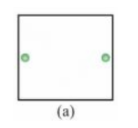The axes of symmetry is as shown :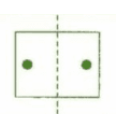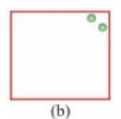The axes of symmetry is as shown :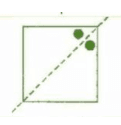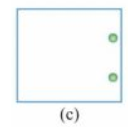The axes of symmetry is as shown :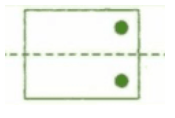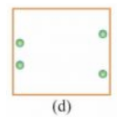The axes of symmetry is as shown :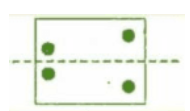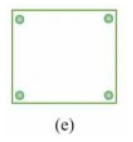The axes of symmetry is as shown :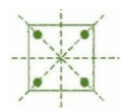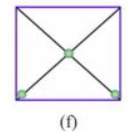The axes of symmetry is as shown :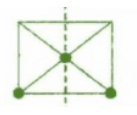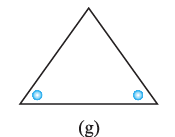The axes of symmetry is as shown :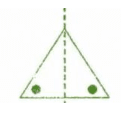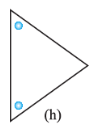The axes of symmetry are as shown :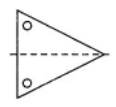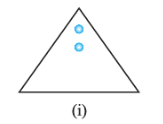The axes of symmetry is as shown :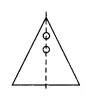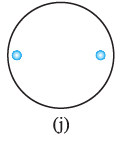The axes of symmetry is as shown :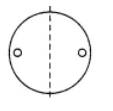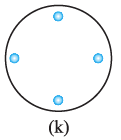The axes of symmetry is as shown :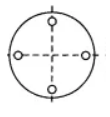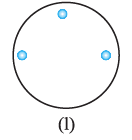The axes of symmetry is as shown :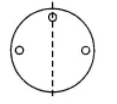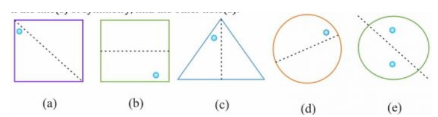The other holes  from the  symmetry are as shown :The complete figures are as shown :(a)   square                (b)triangle            (c)rhombus(d)circle                 (e) pentagon           (f) octagonIdentify multiple lines of symmetry, if any, in each of the following figures: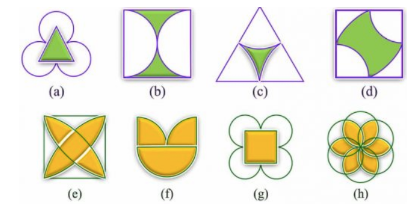The  lines of symmetry of figures are:

(a)There are 3 lines of symmetry. Thus, it has multiple lines of symmetry.(b) There are 2 lines of symmetry. Thus, it has multiple lines of symmetry.(c)There are 3 lines of symmetry. Thus, it has multiple lines of symmetry.(d)There are 2 lines of symmetry. Thus, it has multiple lines of symmetry.(e)There are 4 lines of symmetry. Thus, it has multiple lines of symmetry.(f)There is 1 line of symmetry.(g)There are 4 lines of symmetry. Thus, it has multiple lines of symmetry.(h)There are 6 lines of symmetry. Thus, it has multiple lines of symmetry.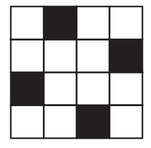The figure with symmetry may be as shown :Yes,there more than one way .

Yes,the figure be symmetric about both the   diagonals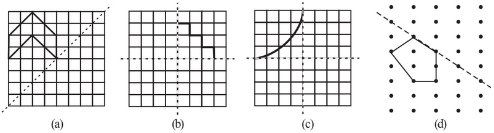The  complete  shape  symmetric about the mirror line(s) are :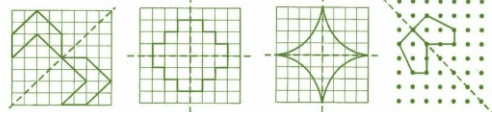(a) An equilateral triangle       (b) An isosceles triangle           (c) A scalene triangle
(d) A square                           (e) A rectangle                           (f) A rhombus
(g) A parallelogram                (h) A quadrilateral                      (i) A regular hexagon
(j) A circle

(a) An equilateral triangle

The number of lines of symmetry  = 3(b) An isosceles triangle

The number of lines of symmetry  = 1(c) A scalene triangle

The number of lines of symmetry  = 0(d) A square

The number of lines of symmetry  = 4(e) A rectangle

The number of lines of symmetry  = 2(f) A rhombus

The number of lines of symmetry  = 2(g) A parallelogram

The number of lines of symmetry  = 0The number of lines of symmetry  = 0(i) A regular hexagon

The number of lines of symmetry  = 6(j) A circle

The number of lines of symmetry  = infinite(a) a vertical mirror

(b) a horizontal mirror

(c) both horizontal and vertical mirrors

(a) a vertical mirror   : A,H,I,M,O,T,U,V,W,X and Y

(b) horizontal mirror : B,C,D,E,H,I,O and X

(c) both horizontal and vertical mirrors : H,I,O and X.

The  three examples of shapes with no line of symmetry are :

2. Scalene triangle

3.Parallelogram

Question:10.(a)  What other name can you give to the line of symmetry of

an isosceles triangle?

The line of symmetry of an isosceles triangle is median or altitude.

Question:10.(b) What other name can you give to the line of symmetry of

a circle?

The other name we can give to the line of symmetry of a circle is the diameter.

## NCERT solutions for class 7 maths chapter 14 symmetry topic 14.3 rotational symmetry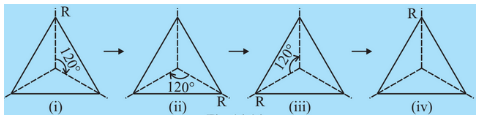An equilateral triangle has rotational symmetry at  angle. The order of the rotational symmetry for an equilateral triangle is 3.All the triangles look same when rotated by . Thus, there are 4 positions at which the triangle looks exactly the same when rotated about its centre by 120°.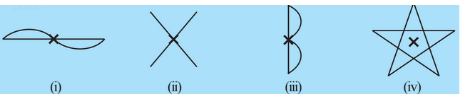Among the above-given shapes, (i),(ii) and (iv) have rotational symmetry about the marked point.

Solutions of NCERT for class 7 maths chapter 14 symmetry-Exercise: 14.2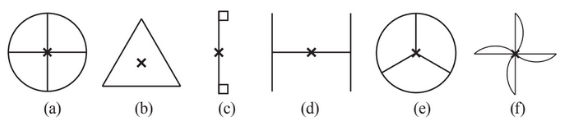Among the above-given shapes, (a),(b), (d),(e) and (f) have more than one rotational symmetry.

This is because, in these figures, a complete turn, more than 1 number of times, an object look exactly the same.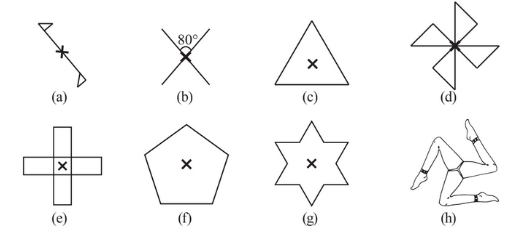(a) The given figure has rotational symmetry about  so it has ordered as 2.

(b) The given figure has rotational symmetry about  so it has ordered as 2.

(c) The given figure has rotational symmetry about  so it has ordered as 3.

(d) The given figure has rotational symmetry about  so it has ordered as 4.

(e) The given figure has rotational symmetry about  so it has ordered as 4.

(f) The given figure has rotational symmetry about  so it has ordered as 5.

(g) The given figure has rotational symmetry about  so it has ordered as 6.

(h) The given figure has rotational symmetry about  so it has ordered as 3.

CBSE NCERT solutions for class 7 maths chapter 14 symmetry-Exercise: 14.3

The  two figures that have both line symmetry and rotational symmetry are :

(i) Equilateral triangle

(ii) Regular hexagon

Question:2(i) Draw, wherever possible, a rough sketch of

a triangle with both line and rotational symmetries of order more than 1.

Line of symmetry as shown below :The rotational symmetry as shown below :Question:2(ii) Draw, wherever possible, a rough sketch of

a triangle with only line symmetry and no rotational symmetry of order more than 1.

A triangle with only line symmetry and no rotational symmetry of order more than 1 is isosceles triangle.Question:2(iii) Draw, wherever possible, a rough sketch of

a quadrilateral with a rotational symmetry of order more than 1 but not a line symmetry.

A quadrilateral with a rotational symmetry of order more than 1 but not a line symmetry is parallelogram.Question:2(iv) Draw, wherever possible, a rough sketch of

a quadrilateral with line symmetry but not a rotational symmetry of order more than 1.

A quadrilateral with line symmetry but not a rotational symmetry of order more than 1 is kite.Yes. If a figure has two or more lines of symmetry,than it  should  have rotational symmetry of order more than 1.

Question:4 Fill in the blanks:

 Shape Centre of Rotation Order of Rotation Angle of Rotation Square Rectangle Rhombus Equilateral Triangle Regular Hexagon Circle Semi-circle

The given table is completed as shown:

 Shape Centre of Rotation Order of Rotation Angle of Rotation Square intersection point of digonals. 4 Rectangle Intersection point of digonals. 2 Rhombus Intersection point of digonals. 2 Equilateral Triangle Intersection point of medians. 3 Regular Hexagon Intersection point of digonals. 6 Circle centre of circle infinite any angle Semi-circle centre of circle 1

The quadrilaterals which have both line and rotational symmetry of order more than 1 are :

1. Rectangle

2. Square

3. Rhombus

After rotating by    about a centre, a figure looks exactly the same as its original position, then it will look symmetrical on rotating by  All angles are multiples of .

We can observe that the angle of rotation is the factor of ,then it will have rotational symmetry of order more than 1.

(i)  is a factor of  so the figure having its angle of rotation as  will have rotational symmetry of order more than 1.

(ii) is not a factor of  so the figure having its angle of rotation as  will not have rotational symmetry of order more than 1.

## NCERT Solutions for Class 7 Maths- Chapter-wise

 Chapter No. Chapter Name Chapter 1 Solutions of NCERT for class 7 maths chapter 1 Integers Chapter 2 CBSE NCERT solutions for class 7 maths chapter 2 Fractions and Decimals Chapter 3 NCERT solutions for class 7 maths chapter 3 Data Handling Chapter 4 Solutions of NCERT for class 7 maths chapter 4 Simple Equations Chapter 5 CBSE NCERT solutions for class 7 maths chapter 5 Lines and Angles Chapter 6 NCERT solutions for class 7 maths chapter 6 The Triangle and its Properties Chapter 7 Solutions of NCERT for class 7 maths chapter 7 Congruence of Triangles Chapter 8 NCERT solutions for class 7 maths chapter 8 comparing quantities Chapter 9 CBSE NCERT solutions for class 7 maths chapter 9 Rational Numbers Chapter 10 NCERT solutions for class 7 maths chapter 10 Practical Geometry Chapter 11 Solutions of NCERT for class 7 maths chapter 11 Perimeter and Area Chapter 12 CBSE NCERT solutions for class 7 maths chapter 12 Algebraic Expressions Chapter 13 NCERT solutions for class 7 maths chapter 13 Exponents and Powers Chapter 14 NCERT solutions for class 7 maths chapter 14 Symmetry

## Benefits of NCERT solutions for class 7 maths chapter 14 symmetry-

• You will learn to identify different types of symmetry and also the order of the symmetry.

• It will make your homework easy as you will find the detailed explanations of all the NCERT questions including practice questions given below every topic in this article.

• You should try to solve all the NCERT questions including examples. If you facing difficulties in solving them, you can take help from these solutions.

• After every topic, there are some practice questions given in the textbook to give you conceptual clarity. In NCERT solutions for class 7 maths chapter 14 symmetry, you will find solutions to these practice questions also.

Happy learning!!!